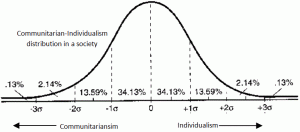Search
•Rana Basheer

# Marcum the Extremist

“Moderation is the center wherein all philosophies, both human and divine, meet.” – Benjamin DisraeliWhile being bed bond and recuperating from a mild viral infection, I had this epiphany regarding a method to measure the influence of extremist elements in a society. My idea was to take a political affiliation poll similar to the one conducted by Gallup and extrapolate that information to see how much percentage of the population has extremist tendencies.

### Who is an Extremist?

According to the American Heritage Dictionary, any person who advocates or resorts to measures beyond the norm is termed as an extremist. This is an interesting definition that lends itself to some mathematical chicanery. So let us start by defining an ideal society and then come up with a method to look for extremist among us. I can’t help but feel that this is sounding more like a statistical McCarthism…..

### Ideal Society

If we analyze any of our recent hot button issues such as gay marriage, abortion, economy, health insurance etc. there are two independent schools of thought pushing and pulling our national dialogue. One school advocates that the individual rights trumps any societal concerns and another one that puts a premium on community. We tend to associate the former with the Individualism while the latter is termed as communitarianism. If we draw a straight line with communitarianism on the left end of this line and the Individualism on the right end, then a person’s affiliation towards any hot button issue would be a point somewhere in this line. One can safely assume that if we aggregate this communitarian/individualism value over a population and plot its histogram it would look like a bell curve as shown below.Communitarian-Individualism distribution in a society

This is not a stringent assumption as communitarianism/individualism tendency of a person along dimensions such as economy, culture etc. are the cumulative effect of several independent small factors such as region, religion, friends, family history, age, social class etc. Even if these individual factors may have different distribution than normal (bell curve), the cumulative effect will tend towards a normal distribution due to  central limit theorem. In the above bell curve around$2\sigma$

### Extremist are the scum in an ideal society

While browsing various publications online about the definition of an extremist, I came across an interesting article titled “Norms in the Global Struggle against Extremism“. In this article, extremism is a quality control problem of a society. They employ the popular six sigma deviation metric to measure if an individual’s view has stepped way outside the norm. On an average this would imply around 3.59 individuals will have extremist view in our above ideal society with a population of 10 million. My plan is to extend this six sigma deviation into multiple political issues and come up with a single factor that accounts for the measure of extremism in a society.

The above communitarian/individual scale could be extended to any number of political issues. David Nolan, one of the founders of American Libertarian Party developed a 2 dimensional chart called the Nolan Chart. His chart plots an individual’s communitarian/ individualist affinity along Economy and Cultural dimensions. For our analysis let there be M such mutually independent issues that are of concern. Let the affinity of a citizen along these dimensions is represented by a multi-dimensional random variable$X=\left[ X_1, X_2, \cdots ,X_M\right] \in \mathbb{R}^M$$X_i \in \mathbb{R};i \in {1,2,\cdots , M}$

gallup data, M=1. Whatever may be the number of dimensions that was used to quantify a person’s political view, we can safely assume that X follows a multi-variate normal distribution (think of bell curves in each dimension).

Since my primary interest is in detecting the extremist in this communitarian/individualist axis, I will apply a transformation that will take the above M-dimensional data of values and transform into a single dimensional positive value as$Y = \left( \frac{X_1^2}{\sigma_1^2} +\frac{ X_2^2}{\sigma_2^2} + , \cdots , + \frac{X_M^2}{\sigma_M^2} \right) = \sum_{i=1}^M{\frac{X_i^2}{\sigma_i^2}}$$\sigma_i$$i$

For Nolan chart, the above transformation has essentially wrapped the 2nd, 3rd and 4th quadrant into the first quadrant. If we assume that$X_i ; i \in \left\{ 1, 2,\cdots , M \right\}$$Y \sim \chi^2(M,\lambda)$$\lambda$$P\left(Y\le y\right)=1-MarcumQ\left(\sqrt{\lambda},\sqrt{y},M\right)$$MarcumQ\left(\cdot,\cdot,\cdot\right)$

Marcum Q function. Therefore based on our definition of an extremist, the proportion of extremist in a society is given by

%Extremist =$100\times 2 \times P\left( Y > \left[\frac{3\sigma}{\sigma}\right]^2\right)=200\times MarcumQ\left(\sqrt{\lambda},3,M\right)$

### Extremists in US Political Landscape

Let’s try to look for the percentage extremist in our democratic setup based on the Gallup data. They polled people on their political affiliation as falling into either democrat, republican or independent. Additionally, independents were sub-classified into democrat leaning or republican leaning. Based on my initial assertion that in an ideal society$2\sigma$$\sigma=1$

The first task was to find the mean and variance of a normal distribution that would satisfy the polled data. Subsequently the$\lambda$$lambda= \frac{\mu^2}{\sigma^2}$On an average US had around 2.3% of population with extremist views. Right after presidential elections in 2012 and 2008, the percentage with extremist views noticed a spike. During the turbulent times of 2006-2009 the extremist percentage saw a lot of fluctuations. I will try to plot the similar data for other countries as and when I get my hands on it. If you are interested in extending this theory please feel free to download the matlab code and the data here.

7 views

See All